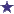# How much of a 1:1000 stock is needed to make a 360ml of a 1:2400 solution?

by Georgette
(Charleston, SC, USA)Pharmacy Ratio / Dilution Question

How much of a 1:1000 stock is needed to make 360 ml of a 1:2400 solution?
Also, how much water is required?

### Comments for How much of a 1:1000 stock is needed to make a 360ml of a 1:2400 solution?

Average RatingRatingby: Anton So, we are given "1:1000" stock. This is equivalent to 1/1000. We want to follow the following equation to solve this question: Amount of strength req'd Stock Req'd = -------------- X Volume Req'd stock req'd So, we plug in the variables to see what we come up with: 1/2400 ------ X 360ml = Amount of Stock Req'd 1/1 1/2400 0.15ml = -------- X 360ml 1/1 The final equation we use in this question is the following: Water Req'd = Volume req'd X Stock Req'd 360ml X 1.5ml = Water Req'd 54ml = Water Required